Select Page

Abraham represents the right side.  Isaac represents the left side. This is not good and evil. It is mercy and judgement. It is day and it is night, one day.  This is what the Zohar tells us. It also tells in that Isaac eyes were not just dim, but totally blind and he was in complete darkness. So, the left was completely blind and out of complete darkness came the blessing for Jacob. The new dawn arose out of the darkness. A metaphor or a prophecy?

A few weeks back Sharon asked what happens when 5778 passes? You do not want to know the answer, at least not while there is still hope. I cannot conscionably tell you what I believed would happen while we are still possibly in the process of escape.  Given that the year we thought to be 5778 has come and past, we might already be in that end-time scenario. Yet, more than 2 years ago I was given permission to reveal a series of equations, methods, technology and codes to allow for either a comfortable reset or better yet, a complete escape.  And the floodgates on those revelations are still open, which means we are still in Neilah and the Ark is still open. Certain important prophecies did come true in their appropriate time and the chances were good for us, yet evil had taken such a strong foothold in our world that Man could not see them. What happens in the next few weeks will determine the fate of the gates and let us know which scenario Man has chosen. I do not believe that it is my place to lay it out for you. It is my place to keep processing these revelations as fast as I can.

As Rabbi Alon Anava hinted at in his video that Sharon shared with us, anything that we can all do to keep the gates propped open will help. He also pointed out that David H’Melech became king of all Israel after 37 years, meaning 2 years past the midpoint in his life, which would give us an addition 4 years to 5782 if the midpoint to Moshiach gets shifted. It does not change the cosmic mathematics to 5778, but I will gladly accept the additional time for mankind.  There are parallels to our lifetime too, as David was a great king but only for half the people. It took 7 years before he was that great king for all the people. We pray for that time to come again and for the Creator’s mercy. In the meantime, there are still many more revelations to be made. For anyone that has not been following this series from its inception, you can find the entire work here.

# Circles

Every infinite slice of a sphere through its center is a circle or disc.  Every ring around the sphere is a circle. Time and time again, the underlaying mathematics of the Torah and the Cosmos relate to simple geometry.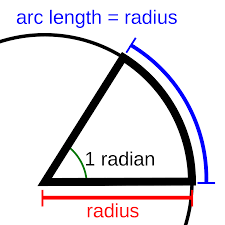Regardless of how we see circles, radians are how scientists prefer to see them. A radian is the angle made when the radius of a circle is wrapped partially around the circle. The angle measured out by the wrapped radius is always 57.295779o regardless of the size of a circle. The beauty of a circle is that it has one primal shape that cannot vary. Being independent of measure it is dimensionless.

A radian is equivalent to a single (1o) degree times 180/π or 57.295779o.  The formula calls for 180 to be used because basic geometry tells us that there are 2πr radii in each circumference, and each circle has 360o, hence there are πradii in every half circle of (360o/2) or each 180o.

While 57.295779o is oddly reminiscent of 5729 and 5779 with 50 years (1 Jubilee year) between them, we will shortly see how odd that really is.

The angle 180o is half a circle. It is also a straight line.

Pi(π), like Phi(φ) are dimensionless numbers because they are primordial ratios and completely independent from various forms of manmade measures, like time, length, mass or current. So, measuring out radians from Pi(π) ties them to that dimensionless realm beyond man’s assumptions about physics.  Because of the simple formula π/180, the angle 18o = .3142, or .314159 or 3.14159159265358…/10. As we said, this is independent of measure, so the circle and radius can be as large as you want.  We should keep in the back of our minds that the number 18 was extremely important to Tesla and has been to us throughout this journey of revelation from 18-digit sequences, some that repeat endlessly, to the 18 words in the 13 Attributes and so much more.

We will explain the significance of that now, and it will come up again at the end of this chapter in a much more profound way. Meanwhile, as illustrated numerous times already, 3142 is the small gematria of “I am that I am (אהיה־אשר־אהיה)” found at Exodus 3:14 and it is also the value of the two most powerful Names, Ehyeh (אהיה) and the YHVH (יהוה), when expanded into 3 dimensions (iterations) together.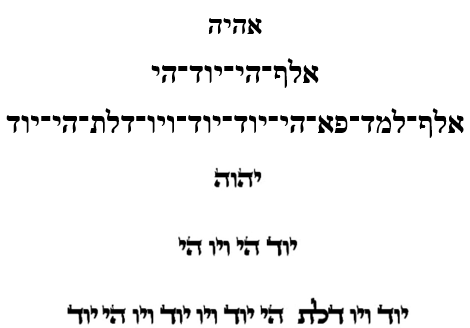Another profound Torah connection that we simultaneously revealed deals with the 42-Letter Name and its first line of value 506 along with its small gematria value0 .123049, since its complete value is 3701 and (3701/506) = 7.31422, while 9/.123049 = 73.1415, or 70 plus Pi(π). Every bit of these equations will soon fall into place. Notice, how the number 9 comes into play so significantly here.

# Sun Cycles

A cycle can be a circle or a wave function or both.

As we explained in chapter 28, by dividing numbers by the value of the sacred cubit/frequency (27.5 Hz) it becomes a filter that sorts them into groupings of 10, whose remainders are all based on 9: .090909…, .181818…, .272727…, .363636…, .454545…, .545454…, .636363…, .727272…, .818181… and .909090….. between every 11th marker number.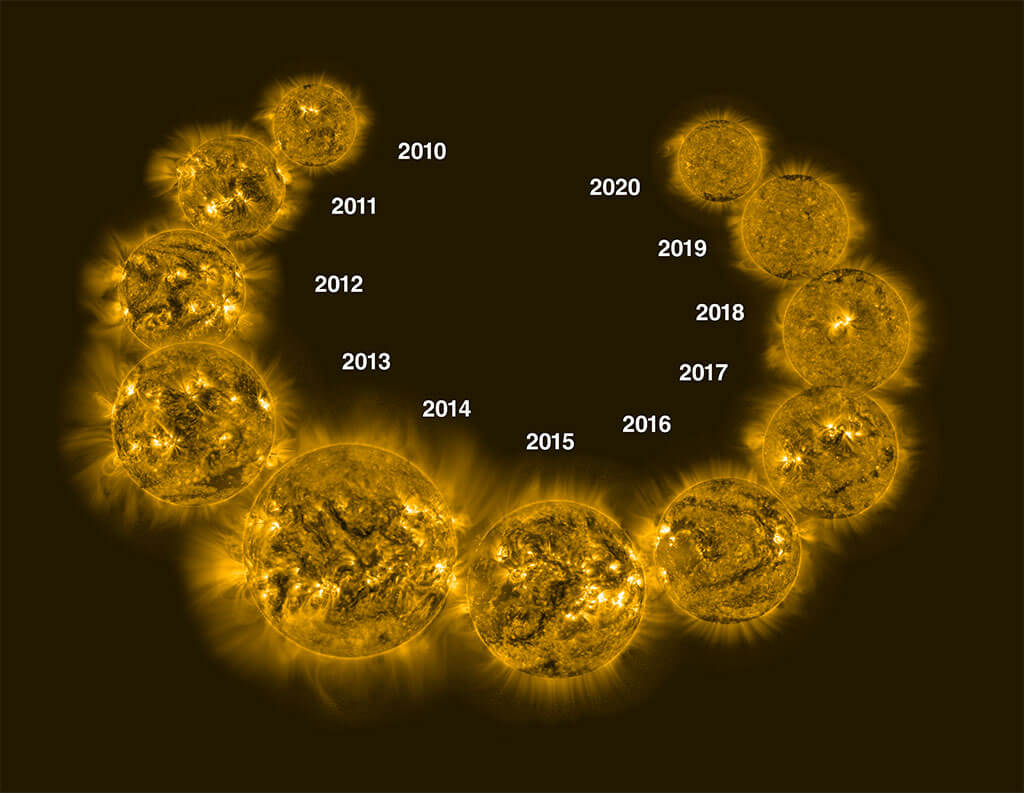Those markers (numbers) are in cycles of 11, like our 11 year solar cycle, starting with 11, 22, 33, 44…. Each one advances the result (11/27.5) by .4, or (.4, .8, .12, .16…) for each consecutive marker. The number 110 is the 10th cycle of 11 years/numbers and has a resultant value of exactly 4, as in (10 x .4) and as in (4 x 27.5) = 110.

As for the 10 bundled numbers within each set of 11, the sum of the results of dividing the consecutive integers by 27.5, starts with 2 for the first set of 10 and advances 4 numbers with every subsequent set (2, 6, 10, 14, 18…). The first observation is that it would appear that there is an affinity for the frequency 27.5 and the number 4 in bundles of 10, not to mention cycles of 11.

### The First Inflection Point

As it turns out, the 5th bundled set of 10 has a 27.5-cycle value of exactly 18, and all 50 (5 x 10) bundled numbers, which corresponds to the 55 (5 x 11) total numbers, have a 27.5-cycle value of exactly 50. We should not gloss over this as all these numbers will get more significant as we go along.  This is very specific inflection point in the scall of all integers divided by 27.5. As the integers increased there resultant quotients total closer and closer to the number of bundled results.  At the 54th integer there are 5 bundles of 10 completed or 50 in total and the sum of the 50 bundled results is exactly 50. Keep in mind that the sum of the 5th of the 5 sets (bundles) of 10 is 18 and 18 is exactly 1/3 of 54. Moreover, the first 3 of the 5 sets also total 18. This 18 total value is at the 33rd integer, once again tying 18 and 33 together, as we saw in the center of the 42 Letter Name.

Since the 55th integer is part of the set of every 11th number and thus nothing changes with regards to the 50 bundled numbers, this is ostensibly the same inflection point.  For every integer above this inflection point the value of the bundled results gets higher and higher vis-a-vis the number of them. That 5 bundles of 10 should equal 50 is special and that it is an inflection point makes it extraordinary and connects them to the 50 Gates of Binah and the 10 Gates of 50 cubits height and 10 cubits width each in the Future Holy Temple.

We know the number 5 is at the core of the Torah and of Phi(φ) and of 27.5, as both 5 x 5.5 and the limit (5.0) to each bundle of 10 of the 27.5-cycle (x/27.5) results or residues. We know that we are talking about the set of all numbers taken sequentially, one decimal place at a time in the base-10 system of mathematics. We just saw the connection between Pi(π) and 18. The number 50 keeps coming up and it continually relates to the 50 Gates of the hyperspace dimension of Binah, meanwhile 55 is not only 2 x 27.5, but the height of the Pyramid’s entrance in feet, 55’. It all bears keeping in mind, especially the part that the 11-number cycle plays in all this.

Scientists are still perplexed why by all observations the Sun conforms perfectly to an 11-year cycle of activity. The 11-year solar cycle starts off with a flipping in Sun’s magnetic field.  The Sun’s polarity switches from N to S and S to N every 11 years. This kicks off a Solar Minimum with few sunspots and coronal emanations. Over the 11-year cycle, those sunspots, coronal emanations, and solar activity build to a Solar Maximum halfway (5.5 years) through the cycle before waning again towards the next cycle. That is its inflection point. Some maximums are more powerful than others and their effect on Earth more profound, yet the cycle never changes, like clockwork.  Given the state of technological dependence on Earth, a very power solar maximum could have devastating consequences for humans, and yet just be part of the Sun’s natural cycle.

# The Odd Matter of the Temperature of CreationEarlier in this series and in prior articles we covered extensively the connect between how the 3 temperature scales interact and form the base measurements of the Future Holy Temple, etc. We also covered most notably how the Sun’s surface temperature is 5778 K and even the slightest variation from that would eradicate or preclude life on Earth as we know it.  So given what we know about the 27 fixed positions in the Essential Cube of Creation at the core of Creation and that 45 is the numerical value for Man, both literally and spiritually through the aspect of the YHVH (יהוה), how odd is it that the average cosmic microwave background radiation (CMB), the leftover radiation from the so-called “Big Bang,” is 2.7 K, or –270.45o C. or –454.81o F? And even more so, as we will explore shortly, that 481 is the height of the Pyramid?

When all the space, dark space, matter, dark matter, counterspace, energy, dark energy and everything else you can think of is added up and a thermometer is stuck in it, it all works out to is 2.7 K, which is also –270.45o C. and also –454.81o F depending which scale you use.

Interesting that the universe chose 9’s again (27, 45, 81).

# Nations are Born

The first 99 numbers, corresponding to Abraham’s age (99 years) when Isaac was miraculously conceived, represent 9 cycles of 11, nine (9) cycles of the Sun’s polarity flipping like clockwork every 11 years. This equals a total 27.5-cycle value of 162 for the 9 bundles of 10, which works out to 9 x 18. Those 9 bundles of 10 equal 90 years, which is equivalent to Sarah’s age when she miraculously conceived Isaac with Abraham.  Women’s cycles are usually on a lunar cycle, but the Torah goes out of its way to tell us Sarah was no longer menstruating. Now we find out that Abraham and Sarah, whose name has the gematria of 505, were on the solar cycle. Was Joseph also on that solar cycle after all he lived 110 years?

In Kabbalah, the moon and lunar cycle are considered Malchut or derived from the physical, while the Sun is Zeir Anpin and is derived from the spiritual. The Torah was sending us a message while showing us its Creator was well aware of our Solar cycles.

The sum of the numbers (integers) from 1 – 100 is 5050, and Sarah (505) gave birth to her only child when Abraham was 100.

We can already see the Creator purposely weaving clues of these extraordinary cycles into this key part of the Torah narrative, and to that we can add that Ishmael was 13 at the time of this miraculous conception and that 13 plus 162 (the 9 bundles of 10) equals 175, Abraham’s age upon his death. We keep saying miraculous because the Torah specifically tells us so.

Isaac on the other hand lived for 180 years.  If we sum up the 9 values of the set of the 11th years within those 99 integers (years) the total is exactly 18. Therefore, adding those 18 to the 9 bundles of 10 that totaled 162, we get a complete 27.5-cycle value for those 99 years of 180, Isaac’s lifespan. Somehow, the Torah has just taught us about solar cycles and the simple formula π/180 for radians and circles, including the angle 18o = .3142, all weaved into the narrative that began in Genesis chapter 18.

Moreover, that formula of π/180 makes 1o = .0175 radians, converting Abraham’s age (175) and Isaac’s age (180) into an inverse relationship with Pi(π) and One(1) and the circle.

In Genesis 18:18 God says, “Abraham is about to become a great and mighty nation, and through him all the nations of the world will be blessed.” This year the 8th day of Channukah falls on the 18th of December in the solar calendar. The Abrahamic Accords are up in the air. The fate of the 45th President will decide the future.

These cycles of 9 and 11 make us wonder about 9-11 that occurred 9 months before the end of the 1370-year period prophesied to end the Arab reign over the Israelites, which began with Mohammed’s death on 6/08 632 CE. Ishmael, the forefather of the Arabs, lived to the age of 137.  God told Abraham that Ishmael would give birth to 12 princes and give rise to a great and populous nation. That prophecy/promise was kept.

Of course, much of this math works out as it does because every 4 cycles of 27.5 is 110 (years) or 10 cycles of 11, which was not overlooked in the Torah either since Joseph as the 4th Patriarch lived 110 years, and 110 is the gematria value of Nes (נס), miracle.

### The Sacred Frequency and the Circle that Turns Time

So, when the sacred frequency of 27.5 is applied, all numbers have a residue or binding connection to the number 9, except every 11th one, a cycle to which our Sun strictly adheres. This makes the 10 bundled numerical elements extraordinary, and it makes the exceptional series of every 11th number extraordinary as well.

This is a rhythm. It is a regular heartbeat for both the Sun and mathematics.

Stop. Think about that for a moment.

If we were to take them as a series of 10 angles (9o, 18o, 27o, 36o, 45o, 54o, 63o, 72o, 81o, and 90o), they would sum to 495o or 1 full circle (360o) plus an additional 135o turning further around it. This is 1 full circle plus an additional 37.5% of a circle. Thus, the angle 495o of every bundle of 10 represents 1 and 37.5% circles or 1.375% circles, and that translates to 2.75π radians.

The turning circle in increments of 9o occurs in two sequential parts (9o, 18o, 27o, 36o, 45o), which equals 135o, and (54o, 63o, 72o, 81o, and 90o), which equals 360o.  The two parts are 27.27% and 72.72% of the total 495o. Alternatively, the 3 angles (36o, 45o, 54o) also equal 135o.

If you recall, turning the circle by the Phi(φ) angle, 137.5o, will create infinite parallel pathways around the circle. Now, we are faced with a parallel concept that the sacred cubit or frequency applied to the circle will advance the circle by 2.75π radians at a time, which is 1.375% of the circle. This is the natural heartbeat or rhythm of the Spherical Time bubble, and it matches that of the Sun.

This also means the sacred frequency turns the circle at the Phi(φ) angle. Conversely, the movement of the cosmic clock by 9o in cycles of 10, creates both the Phi(φ) angle at the source of the infinite paths of Spherical Time and also the sacred cubit, which is the resultant 2.75π radians divided by Pi(π) .

### Where has the Torah linked the numbers 9 and 10 for us already?

There are 90,100 occurrences in the Torah of the Yuds (י), Heis (ה) and Vavs (ו) components of the Tetragrammaton (יהוה), which is the sum of all the integers from 1 – 424, as in Moshiach Ben David and also the distance to our the nearest Star to our Sun, 4.24 Light years away. The cosmos are quite poetic, since the nearest star to our Sun would be Moshiach Ben David.

Our DNA too is arranged as a ladder with its rungs consisting of pairs, and similar to the Tree-of-Life there are 10 nitrogenous base pairs in each full turn of our DNA helix, bundles of 10. The 4 base pairs in Hebrew add up to 424.

There are also the 91 doubled words in the Torah, which represent the fusion of the YHVH (יהוה) and Adonai (אדני).

Then there is the word Israel (ישראל), of numerical value 541, the 100th prime number, and whose first two letters (ישראל) squared also equal 90,100, and whose last two equal 901.   As the Ramchal points out 90 is associated with Chochma (Wisdom) in which Solomon built the First Temple, and 100 is associated with Keter in which the Third Temple will be built.

### As the Clock Turns

With the primordial dynamics at work, the sum of the 10 base-9 angles listed above, when each is divided by the sacred frequency (27.5), equals 495o/27.5 = 18o, and the sum of their 10 radians is 3.14159

This is important. That extra 135o angle that wrapped around the circle is .375 or 37.5% of the circle, leaves a remainder of .625 of the 2nd circle, as in (20001375) = 625, which matches the dynamic splitting of the Alef-bet that we mentioned in chapter 28 in terms of the Fibonacci sequence.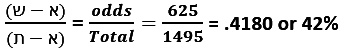That is not why it is important though. The value 1375” equals 50 cubits, the height of each of the 10 Gates of the Future Holy Temple, but that is not why it is important either. And while 1375” or 50 cubits is the height of Noach’s Ark, the volume of that Ark is 45,000 cu3, which may connect it to Man (45) and to the angle 495o that equals 11 x 45, creating an interesting analogy to the 11-year Sun cycles and the 11-bundle cycles of the sacred frequency residues.  We will see an ever deeper connection shortly; nevertheless, this is not why it is important either.

What is significant is that the Phi(φ) angle is 137.5o, and that the Phi(φ) angle is 5 x 27.5 Hz.

As the Phi(φ) angle (137.5o) continually proceeds around a 360o circle, it will generate the Phi(φ) spiral, etching a different place-marker on Spherical Time with every turn, infinitely.  On a single rotation cycle there will be two turns of (137.5o) or 275o within every circle and a remainder of (360o2(137.5o)) = 85o.  The ratio of the full circle to 85o is 360/85 = φ3.

Those two first turns (137.5o) and (137.5o) equal 275, as in our 27.5” sacred cubit and frequency. So a cubit is defined as 1” for every 10o in 2 x Phi(137.5o) turns. The 27.5 HZ would be 1/10th the 2 x Phi(137.5o) turns. You could not use that physically unless you are building a circle with a 57.295779” radius, but it is significant metaphysically. It is significant in Binah hyperspace. It is significant to the vibrational frequency of 27.5 HZ. The connection to Phi(φ) and Pi(π) through the circle is included in every cubit measured out and used in Holy construction, or divinely inspired construction. It is at the core of Spherical Time.

That figure of Phi cubed(φ3) has a special relationship to the Spherical Time bubble in that φ3/√φ = 3.330….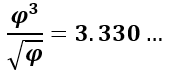It is the same 3330 years that lie between the reception of the Torah at Mt Sinai in 2448 HC and the event horizon in 5778 HC, 66.6 jubilee years later.

Of course, the fact that 5778 is φ18 must play a role as well with Phi(φ) at the core of the Spherical Clock and 5778 at its extreme. As we know, the Sun’s cycle ties in with the clock and  5778 is the surface temperature of the Sun, 5778 K, the same Sun whose longitudinal rotation is 27.275 days, known as the Carrington synodic rotation.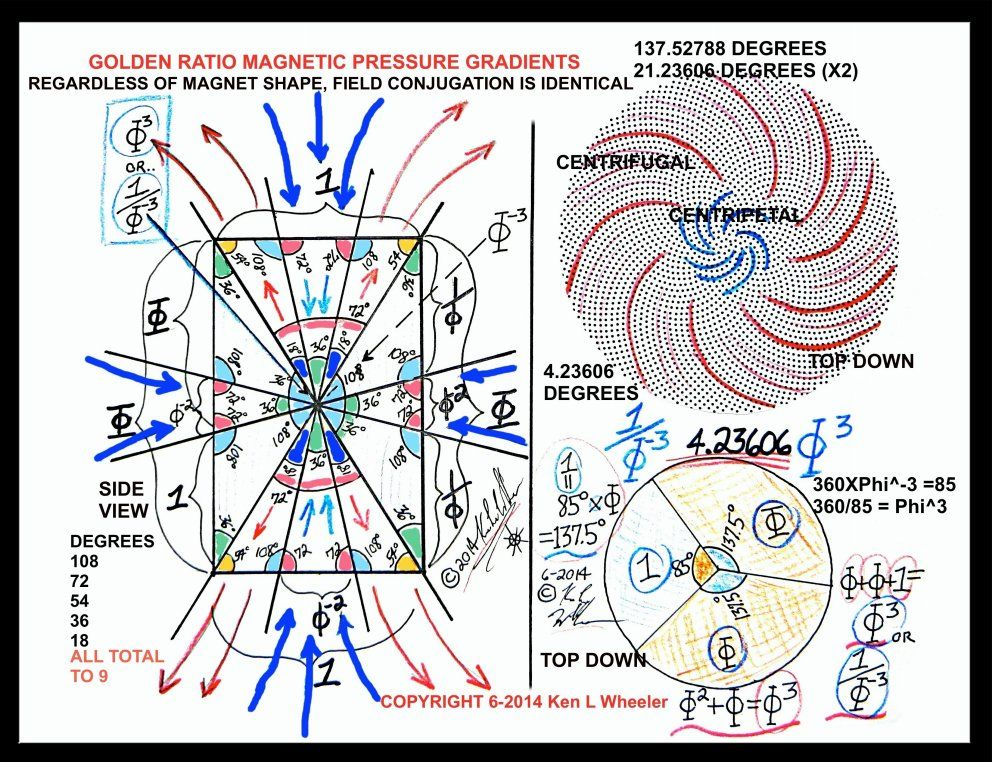# The Sacred Heartbeat

The sacred frequency (27.5 HZ) that gives us a heartbeat of 11 breaths or beats, as in the Vav-Hei(וה) of the YHVH (יהוה), is aligned with the pulse of 11 yet another way. When we divide the set of all numbers by 27.5, it gives us groupings of 10 of every 11 numbers with remainders in groupings of 9’s: .363636…, .727272…, .090909…, …,.454545…, .818181…, .181818…, .545454…, .909090…, .272727…,  .636363….

If instead, we divide by 1.1 (and by any multiple, such as 11) the groupings also similarly and parallelly form in sets of 10 out of each 11 integers, but this time the remainders are all in order: .090909…, .181818…, .272727…, .363636…, .454545…, .545454…, .636363…, .727272…, .818181… and .909090.  Their first group sum is 50, as in Binah, and the set of every 11th integer increases by 10 from 10, 20, 30…

This is the natural order to our universe.

The two frequencies meet at integer 110 since 110 is (4 x 27.5) and (10 x 11).  In fact, there is a special property between these two and it has to do with the interval of the 11th number in each set. For 27.5, it is 4 and for 1.1 it is 10.  The simple equation combining the two results (4 x 10) = 40 reminds us of the 40 weeks of gestation that is 9 months.

Now we can understand why 40 is the age of maturation, the age of Kings, the number of cleansing, the days of rain, and of waiting at Mt Sinai, and the weeks of gestation in the Torah and life. It is even the number of years that King David reigned as King.

The universal constant is derivative of the interaction of the 2 primal frequencies. (1/27.5 + 1/11) = .12727273 or simply .1273, as in 1.273.  Now we can understand the universal harmonic and why it determines the ratio of the square to the circle and the proportions of our planets to one another.

This perhaps explain why those 40 weeks of human gestation are 273 days and why the human menstrual cycle is 27.3 days and thus why there needs to be at least 40 seah of water in a mikvah.

Now, we can better understand why the upper 3 branches of the primordial Alef(א) contain (14 + 11 + 15) = 40 (מ) Triplets, as in the 14 (יד) Triplets of the 42-Letter Name;  the 15 (יה) Triplets of the Shema; and the 11 (וה) Triplets of Bereshit. We will soon understand why the remaining branch, the 72 Triplets is set apart from them. Meanwhile, an equation we looked at long ago seems most appropriate now as we ask about the limits of our Spherical Time bubble: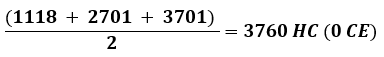1118 is the value of the 15 (יה) Triplets of the Shema. 2701 is the value of the Torah’s first verse, Genesis 1:1 associated with the 11 (וה) Triplets of Bereshit. And 3701 is the value of the 14 (יד) Triplets of the 42-Letter Name.

And speaking of 14, the position at the center of the Essential Cube of Creation, it is also the combination and interval of the two sacred or universal frequencies, as (4 + 10) = 14. That combination increases by 14 every 11th number (14, 28, 42…) Here are some of the other Torah and Creation numbers that correspond to this set or rhythm of 11’s (11, 22, 33…) that we might recognize: 70, 84, 112, 126, 168, 182 (Jacob), 210, 238 (Rachel), 280, 336, 378, 504, 546, 616. Just divide by 14 to see which number within the set it corresponds.

Odd how that the two results of the two sacred frequencies combine such that (4 x 10) + (4 + 10) = 54, the value associated with the full power of the Essential Cube of Creation, and as we will soon see the inflection point.

Instead of their results, if we combine the two frequencies, we get (1.1 + .275) = 1.375 or (27.5 + 110) = 137.5, the Phi(φ) angle (137.5o) that turns our universe.  The 2 primordial frequencies and the circle.

The circle is created by the primordial ratio Pi(π). The two frequencies combine to power the circle to turn at just the right amount to etch Phi(φ) upon it: (1.1 + .275) = 137.5o. They can also combine to close it off, inscribe it (1/27.5 + 1/11) = 1.273.

This all works because the frequency 1.1 is exactly 4% of 27.5. There must be 4 Letters in the Names, 4 sets of Triplets, 4 directions in a plane, and (1+2+3+4) will always equal 10.

As we are about to see, (27.51.1) = 16.5 is also meaningful. Its results are equivalent to (10 4) = 6.

### An Abstract Way of Seeing the Two Primal Frequencies

Yes, to most of the world what we have been studying is extremely abstract already, yet abstractions open our eyes even further. The more we stay tied to the physical and the concrete the less we understand.  We can break down the 27.5 primal frequency into two parts and thus incorporate the other primal frequency 1.1 into it: 1.1(1 + 1.5) = 2.75 and 1.1(10 + 15) = 27.5. If it makes it easier to visualize why we are breaking up 2.5 or 25 into two components think of it as 1.1(a + b) = 27.5 and you can see that 25 may indeed be more that a single solitary number. Indeed, 15 is (יה) and 10 is (י) and 1 is (א) and 1.1 is (וה) so 1.1(a + b) = 27.5 can be (וה)*(יה) + (וה)*(י) = 27.5 or (וה)*(יה) + (וה)*(א) = 2.75

Since we still do not comprehend those fields properly, let us return to the numbers, 1.1(1 + 1.5) = 2.75, and multiple them out, (1.1*1) and (1.1*1.5), and then take the square roots of their products: √(1.1*1) + √(1.1*1.5) = 2.33333210. Well, this is 2 1/3, but is it more?

The ratio of the sacred cubit to the foot, or the sacred frequency to the clock of 12 is 27.5/12 = 2.291666 and (2.333332102.291666) = .041666 or about .042. Nevertheless, the ratio of the Pyramid’s height, 210 sacred cubits to the circle is 210/360 = .58333, or (1 – .41666). Moreover, the average letter value of the 64 Triplets that form the 8000-value square within the 72 Triplets is exactly 41.666.  We will see momentarily that this is not a coincidence. By the way, 1/.041666 = 24.00032.  How many hours in a day?

### The Heart Beat Up Close

The kabbalists would often clasp your hand while they are greeting you.  What they were doing was reading the subtleties in your pulse.

The two frequencies that resonate with the number 9, run side by side, 27.5 and 1.1 Remember, when you hear frequency you should picture sine waves, a number of crests in a single unit. In this case 27.5 and 1.1, with the 1.1 wave 25 times longer (from crest to crest) than the 27.5 one. The 2 of them will be out of sync, but line up or crest at the exact same moment every once in a while. It works out to 2 pulses of 27.5 for every 50 of 1.1.  Some one out there is probably thinking that is 2 senators for each of the 50 states equals 100, but that is probably just coincidence.  Nonetheless, mathematically that works out to (2 x 27.5) = (50 x 1.1) = 55. At this point, the wave height is at it maximum, twice its normal crest.

Both primal frequencies beat every 11 integers and sync up every 5 groups of those beats. What does this mean?

As we divide by 1.1, the results get incrementally closer to a whole number every 11th integer, this is one beat. Those whole numbers are in increments of 10. As we divide by 27.5, the results get incrementally closer to a whole number every 55th integer; this is one pulse. Those whole numbers are in increments of 2. Every 11 integers the results get incrementally closer to a whole number when multiplied by 10; this is one beat. Those whole numbers are in increments of .4, so when multiplied by 10 give us 40.

Therefore, while the heart beats every 11 integers for both frequencies or scales, 5 simultaneous beats each in every 55, or 10 together, there is only one pulse of the 27.5 frequency for every 55 integers.

Now, there is a subtler beat that naturally runs through each of these two frequencies and it syncs with the two primal frequencies at a select natural interval.  They all meet at 55 beats for the 3rd pulse, 5 for the 1.1 frequency and 5 for the 27.5 frequency. This third beat is the foundation beat of the other two. It is based on 9’s and is strictly constructed of the sequential integers divided by either 1.1 or 27.5.

These two separate yet parallel pathways form bridges to one another, besides every 11th rung or subtle beat and have certain equilibrium points that are quite extraordinary. For example, when the two results are added they get closer and closer to the original integer until the number 54, which works out to exactly (54/1.1 + 54/27.5) = 54, the representative number of the Essential Cube of Creation. This is the inflection point. Beyond 54 the numbers get further and further away.

### Phase Points

While 55 is the first of the known phase points of the scales/cycles, the cumulative sum through the 55th integer of the 1.1 scale is exactly 1400, as in position 14 at the center of the same Essential Cube of Creation. What deepens this connection is that 55/1.1 = 50, the letter value corresponding to that 14th position.

At the 10th pulse of those 11 beats, corresponding to integer 110, the cumulative total for the 27.5 scale is 222 and 5550 for the 1.1 scale, or 5772 cumulatively for both. This is the 2nd phase point. While 222 = (60005778), (222/1.1) = 201.8. Go figure! Yet another linkage between 5778 and 2018 and it has to do with the phasing of the primal frequencies or heart beats of our universe.

The value 5772 is also Euler’s Constant (.5772156…)

Go figure, it has to do with the 110 years of Joseph’s life. And speaking of 6000, or the end of the 6th millennium, (5772 + 6) = 5778 and while (2+2+2) = 6, (2 x 2 x 2 x 5 x 5 x 5) = 1000.

Do not forget what we mentioned earlier, that (27.51.1) = 16.5 and its results are equivalent to (10 4) = 6.  What we meant was that (110/1.1110/27.5) = (10 4) = 6.

And speaking of Joseph (156), the sum of the values of both scales together for the 165th integer, as in the midpoint score lines (165 cu) in the bases of the Pyramid, is 156. This leads to the sum of the cumulative values of both scales through 330, as in the Pyramid’s base in sacred cubits, being exactly 312, or (2 x 156), the measure of the Outer Courtyard of the Future Holy Temple.

Abraham lived 175 years and the cumulative sum of the 1.1 scale is 14000 for the 175th integer, again as in position 14 at the center of the same Essential Cube of Creation. Then a very odd things happens when we get to the cumulative sum of all the integers through 275; the 1.1 scale sums to exactly 34500, as in Moses (345), and the 27.5 scale sums to 260, as in the YHVH (יהוה), the cumulative sum of both scales totals 35880 as in Moshiach and Moses’s age at Mt Sinai.

Another inflection point is found at integer 715, whose 27.5 frequency is exactly 26 and where the sum of the two frequencies results are 26 and 650 respectively, or 10 times the value of Adonai, giving us 676, which is the also 262. How amazing that so deep into the interaction of the Primal Frequencies the forces of the universe are designed so that the YHVH (יהוה) and Adonai (אדני) would come together in such a unique way.

You see, this is indeed an inflection point as the combined results gets closer to an exact square of the 27.5 frequency result until we reach 26 and then gets further and further away from it afterwards.  It is a singular confluence in the fields, with the 1.1 frequency being associated with the field and power of Adonai (אדני) and the 27.5 frequency with that of the YHVH (יהוה), two forces eternally entwined and that when properly combined release the power of the Light through squaring, as Rabbi Chaim Vittal of blessed memory advised centuries ago.

We could even say that these are the frequencies of the YHVH (יהוה). This is most significant, perhaps most of all.  The sum of the results for any integer divided by 27.5 and 1.1 then divided by the 27.5 result will always be 26.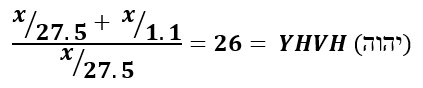Another interesting confluence or phase happens at the 1000th integer, representing Binah. The cumulative value of all the 27.5 frequency results is exactly 18,200, as in Jacob (182) and as in the 1820 YHVH (יהוה) in the Torah.  The cumulative value of all the 1.1 frequency results through that point is exactly 455,000, as in the value (455) of the 3 aspects of Ehyeh (אהיה) times 1000. If this were not extraordinary enough, we find 455 and 1820 side by side in Pascals’s triangle and in the matrix of cumulative numbers, in the 13th order.

1   13  91  455  1820  6188

This has been a lot to take in so far. To visualize it better and to get a better sense of this primordial heartbeat, make a simple spreadsheet dividing all the integers by 27.5 and by 1.1 and then culminating them individually and collectively. There are many more treasures in there as we have highlighted only but a few key ones. The inflection points were most significant as they are not open to interpretation, only to the dimensionless forces of the universe.

# A Lesson in Time from the Pyramid

Our clocks have 12 hours, or 12 time-markers, in them and they are marked around a 360o circle. The height of the Pyramid is 210 cubits, which is 210/360 or 7/12. The base of the Pyramid is 330 cubits or 330/360 or 11/12. The two together equal 18/12 or 1.5 faces of the clock. If we place the 7 as the hour hand and the 11 as the minute hand, the time would be 7:55, as in some of the 755’ bases of the Pyramid. The minute hand, yielding 55 min, also equals the 55’ height of the Pyramid entrance.

In the previous chapter, we highlighted the Spherical Time equations ((5708 + 1948) – 1656) = 6000, and or 27.5(5708 + 1948 1656) = 165,000, uniting the foundation of the Nation of Israel with the Flood more than 4000 years earlier. What is significant about this is that 165 is the small gematria0 of the 42-Letter Name and that it is 1/3rd of 495, the sum of the residues of the frequency of 27.5: (9, 18, 27…90).

The other 2/3rd totals 330, the base of the Pyramid.

When we divide each of those values (residues) by 3 again (9/3/3, 18/3/3 27/3/3…90/3/3) , we get (1,2,3,4,5,6,7,8,9,10), the core of our mathematical system, and a total of 55, or (2 x 27.5).  Funny how 3 plays a role that gives us 55, 165 and 330, or 3 out of the 4 key Pyramid numbers. Of course, the 4 numbers add up to (55 + 165 + 330 + 210) = 760, as in 76.0, ordinal value of the first word of the Torah and the square root of 5778.

Whenever we see 760, we naturally think of 3760 and 5760 and its log, 3.760, but in this case, the number 3 is the clue.

When the 3 highest sefirot (dimensions) Keter (כתר) and Chochma (חכמה) and Binah (בינה) are joined as one, their sum is (620 + 73 + 67) = 760.

We see their connection to the Pyramid dimensions even more through their final letters. When Keter (כתר) and Chochma (חכמה) and Binah (בינה) come together their 3 final letters (ההר) add up to 210, and spell “The Mountain(ההר),” meaning that the 3 sets of front letters (בינ-חכמ-כת) in the 3 highest sefirot equal 550, the sum of (55 + 165 + 330) which is also exactly 20 cubits (20 x 27.5). No one need ask why a Sukkah cannot be higher than 20 cubits anymore, or why the Tower of Truth is 20 stacked cubes equally 210 cubits in total.

Earlier we called these residue numbers/angles base 9 and that is because (09, 18, 27, 36…90) in base 9 convert to (10, 20, 30, 40…100). While this reduced to (1,2,3,4,…10) it also reflects exactly the sum of each set (bundle) of 10 in the 1.1 frequency cycle.

Let us examine what we just said. One-half (1/2) the base of the Pyramid (165 cu) equals 1/3 the phi-angle generating 495o, and the full base (330 cu) equals 2/3 the phi-angle generating 495o. Therefore, 1 base side equals 360o, a complete circle.

### Counterspace

Let us examine this further. It is not just dividing 495 by thirds, but by dividing each of the 10 numbers/angles by 3.  We reduced 495 by 9, or 495/9, to get 55.  First, we reduced a string of 10 consecutive numbers by 27.5 and got their residues, which summed to 495. Then we reduced those by 3 and 3 again, or 9 to get the ordinal values 1 –10. When we divide by 3, we have taken each number, such as 90 and reduced it to 30, leaving a counterspace of 60. In the hyperdimensional realm, by reducing a number, its counterspace does not just vanish like on our calculators. So those ten counterspaces (6, 12, 18…60) that equal 330 are just set aside and have their own field value. When we divide by 3 again from our set of (3, 6, 9…30) that equals 165, we get a new counterspace of (2, 4, 6…20) that equals 110. You could say that the counterspace component is (330 + 110) = 440 out of 495 for each bundle of 10, or 88.888% and the spatial component is 11.111%. We can also say the counterspace component is 440 and the two phases of the spatial components (165 and 55) equal 220.

The counterspace component is the invisible entity that resides with each spatial entity.

# More Abstractions

As stated earlier, 165 is the small gematria of the 42-Letter Name and the two primordial frequencies interact to give us (27.51.1) = 16.5.

Since each of the 4 bases of the Pyramid are scored at 165 cubits, the value 495 represent 3 turns of the 8 scored half-sides or 3/8 of the way around the Pyramid. That equates to .375 of the way around it. Now, while 375 = 165 + 210, the Pyramid’s height, .375 is the extra percentage beyond a complete circle. It takes 3 turns of the Phi(φ) angle (137.5o) turns to complete a circle, and begin a new circle or spiral.  The Phi(φ) angle does not care if it is a circle or a square, and 360o x 210 = 75600, which is the base of the square Pyramid (756’ x 100).  Is this why Tesla circled every building 3 times before entering or each table 3 times before sitting?

We should keep in mind that 165 cu, representative of the small gematria0 of the 42-Letter Name, equals 378’, as in the sum of the 27 positions of the Essential Cube of Creation that is based on 42.

In hyperspace mathematics, we often sum the numbers, especially when they are consecutive, and the first 10 integers sum to 55, or twice the sacred frequency (27.5 Hz), but what about the relationship between those 10 numbers, for example  (2/1, 3/2, 4/3…10/9)? Well, nothing special comes of that, except if we extend it to 13/12. The first 13 numbers sum to 91, and the sum of the 12 ratios between them sum to 14.00…, as in the central position of the Essential Cube of Creation.  Moreover, 27.52, or 756.25, divided by that sum equals 54.0054… firmly representing that same Essential Cube of Creation and leading us to believe the connection was intentional.

Remember Pascal’s Triangle and the matrix we derived from it. The numbers 55 and 91 are both Triangular Numbers, as is 5778, and the other Pyramid numbers, 120, 165, 210 and 330 are all cumulative sums of Triangular Numbers or other cumulative sums.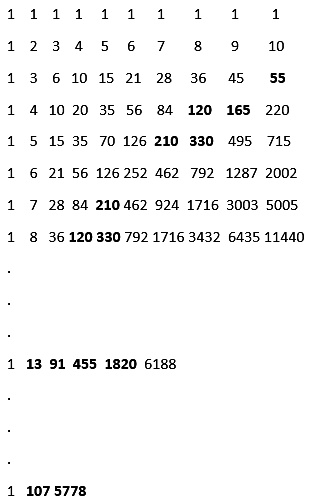If the Pyramid does represent a Spherical Time clock it behooves us to understand it better.

### The Pyramid and Abraham

The base of the Pyramid is 330 cubits. The Spherical Time bubble is set to 5778 years.  The value (or time-length) 5778/330 = 17.50 with a residue or .09090. Thus, the Spherical Time bubble limit divided by the base of the Pyramid is 17.5, the lifespan of Abraham. To be clear, the Pyramid’s height (5775”)/330 cu is precisely 17.5.  The entrance to the Pyramid is 55’ high off the ground and 55’/481.25’ (Pyramid’s height) is .11428571 or 11.42..% of the way up. The value .11428571 = 1/8.75 and 8.75 is 17.5/2, one-half the Pyramid’s height/base ratio.

### A Base of 72

The scored midpoint of each base is 165 cubits long, one-half each side. A cubit is 27.5”, matching the sacred frequency of 27.5 Hz.  The 165 cubit mid-length equals 6 multiples of 27.5.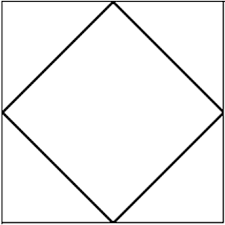If we divide the Pyramid base into 4 equal squares, each side of those squares would be 165 cubits. Each square would be 6 multiples of 27.5 by 6 multiples of 27.5 or 36 multiples of 27.52. Each half of the Pyramid in either direction would have an area of 72 x 27.52.

Using Pythagoras’ Theorem each diagonal of each of those 4 squares would be 233.3452378 cubits long. Now it gets interesting. That diagonal is equal to 27.5 times the square root of 72, or 27.572. If we were to form a square within the base of the Pyramid from those 4 connected diagonals, their perimeter would be 933.3809512… or 4 x 27.572, which equals 11072.

This new square carved out of the diagonals of the 4 corner squares of the Pyramid, has an area necessarily exactly half that of the Pyramid. That area of the inner Square is 54450 cubits squares, and it turns out that  if we divide that by the base of the Pyramid in feet, 756.25’, or in other words by 27.52, it works out to exactly 72. If you are trying to visualize this, the square formed by connecting the scored midpoints of each side of the Pyramid to one another and thus at a right angle to the orientation of the Pyramid, is comprised of precisely 72 squares of 27.5 by 27.5, our sacred cubit times sacred frequency.

As astonishing as this is, if forces us to ask what this means for the 72 Triplets and how they might react or are supposed to react with the sacred frequency 27.5 HZ squared.

Now we can understand why the 8 x 9 Matrix of the 72 Triplets was split into a row of nine and a square of 64 that totaled 8000, or one (3 x 3) square of 9 and one (8 x 8) square of 64.  You cannot create a square of 72 unless you have irrational sides. Each side would have to be √72 or 8.48528137424… units. This is a most telling number 8.48528137424, but not a whole one: twice Moshiach Ben David (8.48); the mile 5280’ plus the fine structure constant, etc 137; plus Moshiach Ben David (424)…

The central 72 square of the Pyramid aligns as two squares, one of 82 and one of 32. The 32 square aligns it with the 9 planes of the Essential Cube of Creation, each a 3 x 3 square.

### Critical Ratios

Another extraordinary property of the Pyramid we need to keep in mind is found in the ratio of its unique area to its perimeter. This ratio of area/perimeter is unique to every imaginable square and varies wildly from square to square. In the case of the Pyramid, though, it works out to 330 x 4 or 1320 cu for its perimeter and 3302 for its area, and the ratio between them is A/P = 82.5 : 1 or (3 x 27.5)/1. The inverse of that ratio is P/A = 1/(3 x 27.5) = .0121212

This brings up another critical ratio, that between the sacred cubit and the number 12. It is this ratio that makes the interaction of the inch, foot, and cubit work out so perfectly with regard to Pyramid.  Why did there have to be 12 sons of Jacob, 12 Tribes of Israel?

Since the central square within the Pyramid is half the area of the Pyramid and since it equals precisely 72 squares of 27.5 by 27.5 then the full base of the Pyramid is 144 squares of 27.52 or a perfectly square grid of 122 x 27.52.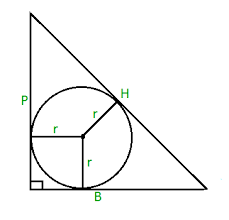Let us go back to the base of the Pyramid, where we see it marked by a crosshairs of 165 to each side and a vertical tower of 210 at its center. Given the angle of ascent and simple geometry the radius of a circle drawn to neatly fit inside that vertical triangle (165210267) is 54. That circle can be made into a sphere and exactly 2 such spheres can be constructed inside the pyramid, one in each half.  Yet if the circle, or ring, were to be generated as a series of rings pivoting on the central vertical column, then we would have a torus of diameter (54 x 4) = 216 cubits, as in the 216 letters in the 72 Triplets. It seems the Creator of the Pyramid really understood geometry, sacred and otherwise.

### Ascension

What are the first steps of the initiate? The initiate must climb up 10 steps, through the 10 sefirot (dimensions). The first is 1 foot high, the second is 2 feet high, then 3 feet high until the last step of 10 feet. At that level, he has ascended 55’ in total and finds himself at the hidden entrance of the Pyramid. It is 55’ straight up a steep 51.843o angle, up a shear, smooth and blindingly white polished face of the Pyramid.  Geometry shows us that that vertical height of 55’ is 70.0 actual feet along that steep face, matching the 70 steps Joseph climbed to meet Pharaoh.

The ratio of actual feet plotted on the face of the pyramid vs vertical feet is approximately 1.273, the universal harmonic once again. Is the question why 70 family members entered Egypt, or why Adam gave David 70 years or why the Pyramid was built to the precise dimensions so that its entrance would be 70.0 feet? Or is it that the universal harmonic times the sum of 1-10 would be 70? The square around the circle of 55 is 70.

### The Pyramid and the Essential Cube of Creation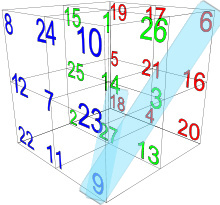The measurement 55 cubits is 126.042 feet, and 165 cubits is 378.13’, the respective values of each plane along with the total positional/ordinal value of the entire Essential Cube of Creation. And 330 cubits is 756, corresponding to the 6 faces of the Essential Cube of Creation. These Pyramid measurements (126, 378, and 756) represents respectively 9 ordinal values per face (or plane); any 3 planes; and the 6 faces of the Essential Cube of Creation.

### Transformation

The first letter of the Torah is the large Bet (ב) of numerical value 2. The letter Hei(ה) within the 42-Letters is a gateway. It was given to Abraham. Hence the Torah is 248 columns of 42 rows. Its numerical value is 5. The two together (2 x 5) represent the 10 sefirot. The two together represent to 70: (2 + 5) x (2 x 5) or (2 + 5)/ (2 x 5). There is also an enlarged Hei(ה) in the Torah, one of the 10 enlarged letters connecting to Binah. It is the last enlarged letter of the Torah.

Operating in 11 dimensions vs 10 helps transform our base 10 physicality to the sacred. The number 5 when multiplied by 11 becomes 55, equaling 2 sacred cubits; 52 becomes 275, or 10 sacred cubits/frequency; 5/2 becomes 27.5, the sacred cubit; 10 becomes 110 or 4 sacred cubits; 45 (the sum of 1 –10) becomes 495, the sum of the 10 base 9 residues of either 27.5 or 1.1.

### By Another Measure

There is another measure that some think was used to build the Pyramid. It is .75 of our 27.5” cubit, so it really only works out (somewhat) because it is derivative of our sacred cubit. Moreover, because our cubit is related to Phi, this other cubit is 52.36 cm or 2 x Phi(φ)2, though the connection to the meter may be partially coincidental. As previously discussed, the ratio of the cube to the inscribed sphere is 52.36%, which is most likely the source of this confluence of measures, more than the ancient Egyptians or occupants of Egypt utilizing the meter.

This shorter cubit would be .75 x 27.5 or 20.625”. Using the figure 20.625”, the height of the Pyramid would become 280.147 cuphi, and the base would be 440.231 cuphi, not exactly whole numbers, yet (440.231 + 280.147) does equal 720.3780, interesting numbers nonetheless. And 55’ would equal 32.018… cuphi, again interesting numbers.

Now, let us recall that the counterspace component of the 495 for each bundle of 10 is 440, and we see that the phi-cubit fulfills the role of the counterspace within the residue of the frequency 27.5. With the help of the phi-cubit, the invisible just became visible.

I am aware that I keep asking you to enter my world of every deeper abstractions, but since the sacred cubit or frequency applied to the circle advances the circle by 2.75π radians at a time, then what we have is 2 sacred cubits plus one phi-cubit (.75) equal the 495o of a circle. This is the circle powered by 9’s and that churns the phi clock.

When measured out together, those 2 sacred cubits and one phi-cubit (.75) equal (2 x 27.5 + 20.625) or 75.625”, reflective of the base of the Pyramid, 756.25’. Like every word, letter and verse of the Torah that serves multiple purposes, so do each measure of the Pyramid.

The Pyramid apothem (267 cu) divided by 165, or half the base, equals Phi(φ), and 165 is the equalization point between the 2 different cubit measures. The value 20.625”, as .75 or 1/4 less than the sacred cubit, fits into 165 eight (8) times, while the sacred cubit, which is 1/3 more than the phi-cubit (royal cubit), fits 6 times into 165. And here is the deepest secret. The value 165 is the numerical value of “The Tree (העץ).”

It would have been very difficult for the builders of the Pyramid to measure out .625 of an inch, but all they would have had to have done was score the ¾ mark on a 1 sacred cubit rod.  Did they really need 2 different cubits, probably not, but they must have had their reasons, as we saw with the 2.75π radians? Did they know that 20.625 was the square root of 425.390625, which is the value of the 8th of the 72 triplets along with the 390,625 words, letters, and verses in the Torah? Obviously not, if the Egyptians designed it.

Since the two cubits represent (1 and .75) in respect to 27.5”, combining or adding the two cubits together is equivalent to multiplying 1.75 x 27.5 or 48.125, as in the height of the Pyramid in feet, 481.25’. Moreover, this also tells us the Pyramid’s height in feet is (17.5 x 27.5), relating it to Abraham who lived 175 years. In Genesis 16:5, Sarah said to Abraham, “Let God judge between me and you!” Sarah lived 48 years less then Abraham.

### The Right Angle

As a number, 165 is 45 years less than 210 years, as in the exile and the height of the Pyramid in cubits and levels, and yet (17.5 + 27.5) = 45.  The entrance, 55’ is 45’ less than 100, and 210 is 90 cubits short of 300. Does this mean anything? Probably not, but 454590 are the angles of the Isosceles Right Triangle.

# The Ratio of the Sacred Cubit and the Foot

As discussed above there is a primordial relationship between the 2 frequencies 27.5 Hz and 1.1 HZ that combine to give us the Phi(φ) angle, etc.  Multiplying together 27.5 and 1.1 gives us (27.5 x 1.1) = 30.25 and there are 3025 inches in the base of the pyramid (27.5 x 110), which works out to 552, or the Pyramid’s entrance height in feet squared. Since (27.5/1.1) = 25, the values 30.25 and 3025 represent graphically (27.5 + 27.5/1.1) and (27.527.5/1.1).

In this one simple product of the two primal frequencies we see the somewhat inexplicable tying together of the sacred cubit (27.5), the foot(12), and the inch(1).

Curiously, the Ascending Passage that leads to the King’s Chamber ascends at an angle of 26o18’ or Phi(φ)2 and its height, along with the height of the granite blocks that plug it, are 2.29 times these ¾ cubits (20.625”) that Egyptologists call the royal cubit. We can call this the conversion stone. The thing about 2.29 is that it is the ratio between the sacred cubit and the foot, 27.5/12, the ratio that makes all the dynamics of the Pyramid work.

### The Mile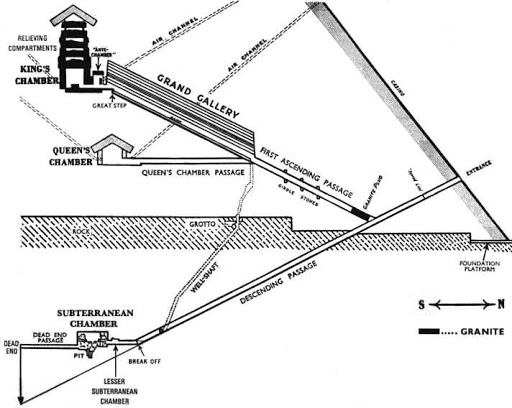What a curious place to hide it. Curious indeed since the length of that passage is 75 royal cubits or 75 of those phi-cubits that are each in turn .75 of the sacred cubit. The conversion stone is at the entrance to the Ascending Phi2 Passage, which continues upward at the same Phi2 angle through the Grand Gallery to the Great Step, where the passage levels off and heads into the Kings Chamber. The distance through the passage from the conversion stone to the Great Step, which is found at the exact midline of the Pyramid, is exactly 280 ft, as in manzipach, the 5 final letters.  And let us not forget that the perimeter of the Pyramid in sacred cubits (330 x 4) = 1320 cubits or ¼ of a mile in feet: (1320 x 4) = 5280.  Then somewhat magically, or at least mysteriously, the perimeter in feet, 3025’, which is also 552, is 3025/5280 = .57216667 of a mile, and (.57216667 -.55) = 2.2916667, the exact ratio of the sacred cubit to the foot (27.5/12).

Someone chose all these numbers very wisely, especially since the mile of 5280’ has only been formulated since the late 16th century, thousands of years after the Pyramid was built. Oddly, the mile was inadvertently chosen as 42 x 330’, since the only intention was for the mile to be 8 English new furlongs of 660’ each, instead of the German furlong of 625 German feet. The 12” foot, though, was already in existence in Egyptian times. How the all-important inch was standardized is still a mystery. Nevertheless, the system was designed/created so that (1 x 12 x 27.5 x 5280) would equal 1742400, which equals 16 x 3302 or 24 x 3302, and the sum of the digits in (1 x 12 x 27.5 x 5280) is 33. The product 1742400 is also 33 x 52800.

Those 280 feet of the contiguous Ascending Phi2 Passage and Grand Gallery are 3360 inches, or 80 x 42 inches. And what may or may not be coincidental is that the 2.29 phi-cubit height equals 47” and the sum of the digits in the Pyramid’s height (210 sacred cubits, 5775 inches, and 481.25 feet) is 47.

Now here is an interesting equation, which may or may not have meaning. Those 3360” and 280’ and corresponding 122.1818 sacred cubits sum to 3762.1818… and 3762.18181/(2.7527.5/12) = 3760.  Let us break that down. The final and grand corridor, utilizing all 3 measures of space, is equal to the Spherical Time bubble, 3760 years, plus 2.18, which is representative of its counterpart Time frame, 2018 years, and moreover, that that 2.1818 difference is equal to the sacred frequency (2.75) less the ratio of the sacred cubit to the foot(27.5/12).

Furthermore, the Ascending Passage portion of this Spherical Time marker is 75 of those phi-cubits that are each .75 of the sacred cubit, or 2 (75’s) as in 2.75.

### King’s Chamber

The King’s Chamber within the Pyramid was constructed to the perfect golden ratio or Phi(φ) proportion regardless of what unit of measure was used. Proportions are ratios and ratios are independent (non-dimensional) of measuring tapes. In the case of the King’s Chamber, the measuring unit that gives the prettiest picture is the ¾ Sacred Cubit measure, which is the 20.625” phi-cubit. The chamber measures 10 x 20 x 11.18 cuphi. The value 11.18, or 55, is reflective of the 15 Triplets of the Shema, 1118.  If we add to those 3 measures the corresponding (7.5 x 15 x 8.385) values using the sacred cubit, we get a total for the 6 measures of 72.0

Nevertheless, utilizing the shorter ¾ phi-cubit only, the chamber has a volume of 2236 cubitsphi.

That is half the story because (2 x 2236) = 4472 and as we have seen before 161 is the value of the Binah Name Ehyeh (אלף־הי־יוד־הי) and 161/√5 = 72.0013 and while 72/161 is .4472, the logarithm of 4472 is 3.650.  And while 1615 = 360.00 or the 360o circle, (161.5 x 20.625”) = 3330” truncated, as in the 66.6 jubilee years between 2448 and 5778. Using a ¾ cubit allows an architect to scale down a design while keeping the same necessary proportions, and it gives him more options to reveal mathematical and time dynamics.

Let us get abstract for a moment and add (2236 + 4472), which is by the way (3 x 2236) or 3 times the volume of the King’s Chamber, to get 6708, the value of the 42-Letter Sword of Moses and the square root of 45. Furthermore, in case you did not notice, 2236 = (2 x 1118), which means that the 42-Letter Sword of Moses and the square root of 45 each equal (6 x 1118), the value of the 15 Triplets of the Shema, which is turn is 6000(φ  – .5). The chamber deep in the heart of the mountain (ההר) of stone is a tribute to Phi(φ). It is constructed out of exactly 100 stones and is connected to the 42-Letter Sword (חרב) of Moses and the 15 Triplets of the Shema. The gematria of both “the mountain” and “sword” is 210, as in the 210 levels and 210 sacred cubit height of the Pyramid.

According to the Zohar and the Arizal, the value of the two letters Hei(הה) in the YHVH (יהוה) have a value of 55 since the first Hei(ה) is the Hei(ה) of Binah with a value of 50, giving them an average value of 55/2 = 27.5

There are 55 verses in the first 2 chapters of the Torah for an average of 27.5.

So now let us follow the clues.  The 4-sided Pyramid is 210 sacred cubits and levels high, or 5 x 42.  There is the 42-Letter Name; the Upper 42 Letters of the Name; the 42 Letters of the Sword of Moses; and the triple 42 of Ehyeh Asher Eyheh; and either the first 42 Letters of the Torah or the Essential Cube of Creation based on 42.

The entrance is 55’ off the ground.  The base side is 330 sacred cubits.  There are 55 Vavs(ו) as initials in the Shema, totaling 330.

### 2:10

Genesis 2:10, the 41st verse in the Torah: “A river flowed out of Eden to water the gardens. From there it divided and became four major rivers.” In this verse we have a depiction of our crosshairs, our alignment of the 5 Essential Elements, and of the Pyramid. All are depictions of the energy flowing out of Binah and downward and outward in 4 directions—a schematic.

### 3:30

Genesis 3:3, which is 18 verses later in the Torah: “But of the fruit of the tree that is in the middle of the garden, God said, ‘Do not eat it, and do not [even] touch it, or else you will die’.”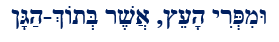The 18-letter phrase, “But of the fruit of the tree that is in the middle of the garden,” is very interesting and revealing, besides the fact that the verse refers to the Tree-of-Life, and to the starting point of the Spherical Time, our physical existence. How long were they in the Garden before Eve set off the clock?

The first word in a Torah portion or verse is often very important. “The fruit of (וּמִפְּרִי)” has a gematria of 336, as in the 336 letters in the 112 Triplets. It also begins with (ּוּמִ) of gematria 46, as in cubit, and it ends in (ְרִי), of numerical value 210. It also ends in (מִפְּרִי) of numerical value 330. The first 3 letters (וּמִפְּ) have a value of 126, or (3 x 42), as in each face and each plane of the Essential Cube of Creation. While the letters (ְוּרִי) have a value of 216, as in the 216 letters of the 72 Triplets, the 4 letters (וּמִרִי) have a value of 256, the same as the Ark of the Covenant. Moreover, the outer letters (וּי)of the 4 (וּמִרִי) have a value of 16, the square root of 256.

Meanwhile, the final 3 letters (פְּרִי) not coincidentally have a value equal to 290, matching the pair (רצ) at the center of the 42-Letter Name. We can be assured it is not coincidental because the square root of 336 is 18.330 and 1833 is the value of the 42-Letter Name until its midpoint at (רצ). Think about the words: “But of the fruit of the tree that is in the middle of the garden.”

That is a lot to pack in one word and it is only the first of the five.The second word “The Tree(הָעֵץ)” must be extremely important. It has a value of 165, as in the distance in sacred cubits to the middle of the Pyramid from each of the 4 midpoints in the bases, where we found the secret of the 72 Names. It also came up for us in one of the Spherical Time equations: (5708 x 27.5) + (1948 x 27.5) – (1656 x 27.5) or 27.5(5708 + 1948 1656) = 165,000, where the simultaneous dates for the birth of the nation of Israel and the date of the Flood were taken into consideration.  Of further note on that, the sum of the digits in 5708 + 1948 = 42 and the sum of the digits in the Flood date (1656) is 18.

The first two words together “The fruit of (וּמִפְּרִי)”  and “The Tree(הָעֵץ)” have a combined numerical value of (336 + 165) = 501, the value of the very next word, the middle word, “Asher (אֲשֶׁר), that is.” This is the same Asher as in the middle word in “I am that I am” (אהיה־אשר־אהיה), the one with an ordinal value of 42, part of the three (3) 42’s in that lofty Name.

When we combine the values of the second and third words (165 + 501) they equal 666.

The numerical value of the word “in the middle of (בְּתוֹךְ)” has a value of 428, while the final word, “the garden (הַגָּן) is 708, as in the numerical value of the Upper 42 letters of the Name.

Now here is a deep and simple secret found in the middle of the garden of Eden at the Tree-of-Life before it was plucked. The initials of the 13 letters in “the tree that is in the middle of the garden,” is Ahava, love (אהבה).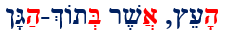The final letters in those same 4 words add up to 360, as in the 360o in a circle and the 4 corners of a square.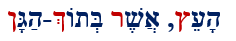The 5 initials in the 18 letters “and love (ואהבה) match the initials in Torah’s first verse.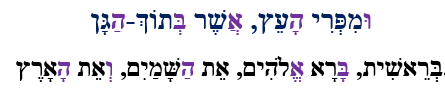From the first 3 words of the verse we can spell out Shema (שמע), while the remaining 8 letters of those 3 words have the gematria of 1118, that of the Shema’s first verse. The final letter in the phrase, Nun(ן) has a numerical value of 50, as in the ordinal value of Shema (שמע), and we should bear in mind that the driving force within that verse that contains the 15 (יה) Triplets is the Upper 42 letters of the Name of numerical value 708 as in (הַגָּן).  If we separate out the (יה), we are left with (ופרץ) in the first two words with a numerical value of 376, the same as Shalom, peace. For all three words it is 577.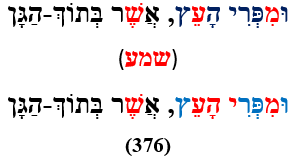The connection to the Shema is even more obvious than that. The full value of the verse is 1488 and the 5 final letters total 370, meaning that the rest of the letters total (1488 – 370) = 1118. The value 370 is that of the 3rd Triplet of the 42-Letter Name (קרע).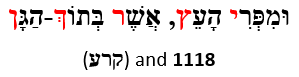Shalom (376) is found again in the 4 letters (העאש).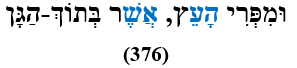Through its initials the phrase “But of the fruit of the tree that is in the middle of the garden,” connects to the 11 triplets of Bereshit; through various means it connects to the three(3) 42-Names and the 14 Triplets of the 42-Letter Name; through the word Tree and also the word “fruit of’ it connects to the 72 Triplets; and through its entirety it connects to the 15 Triplets of the Shema. Moreover, its first words represent all 112 Triplets through their 336 letters.

And there is so much more in there, like the first line of the 42-Letter Name matrix (אבגיתץ) contained in all 5 words, and the total gematria of the verse plus a kolel of 18 for the 18 letters being (1488 + 18) = 1506, and that (1488 – 506) = (100018).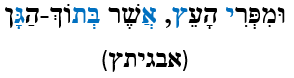Or that the 6th Triplet (יכש) of the 42-Letter Name matrix is found in the verse as well as the 5th (מהש) and 8th Triplets (כהת) of the 72 Names because Moses (משה) used both these Triplets to overpower the Egyptian.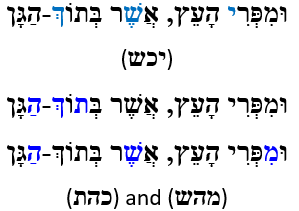Moreover, there is the YHVH (יהוה) in sequential order.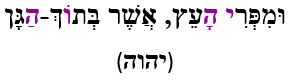Or “V’YHVH (ויהוה) in sequential order of numerical value 32, as in the 32 Paths of the Tree-of-life.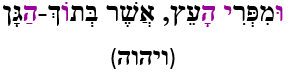And like the 5 final letters (םףץךן) being including within the 18, as if the end was in the beginning, as in Phi(φ)18 = 5778.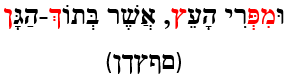And the first 3 final letters (יץר) equaling, 1110 or Alef(א) times 10, leaving the last two final letters (ךן) to total 70 or 1200, depending on whether we are using gematria sofit or not.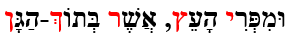And like Asher (אֲשֶׁר) being in the middle if the verse and of Israel (ישראל).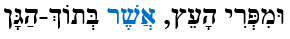And there is more concealed within the 7 letters of the middle of the garden that have a gematria sofit value of 1616.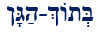The first 3 letters (בתו) have the numerical value 408 as in the missing 408 component letters from the Torah (600,000408) that sum to 2448. The middle 2 letters (ךה) have the value 25 or even 505 in sofit, as in Sarah (505), and the final 2 (גן) have the value 703 as in (ואת־הארץ), the earth from Bereshit 1:1.  When we add the first 3 and the final 2 (גן) we get 5 letters equaling 1111 and 2 equaling 25. The Zohar tells us of the missing 25 letters and ties them to the 25 letters of the Shema’s first verse.

Of, course, the letters (וך) have the numerical value 506 and (תוך) has the value 906 as in the first line (אבגיתץ) of the 42-Letter Name matrix and its last line (שקוצית) with (תו) between them, having the value of the 13th Triplet (שקו)of the same Name. What is in the middle of the garden?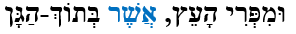According to the Zohar, the distance to Heaven is 500 Parsangs, as in the central letters (שר). The rest of the letters in gematria sofit add up to 2928, the years Solomon built the First Holy Temple.  That was the year 10 became 1, as in (10/1 + 10/2 + 10/3…10/10) = 29.28….

### 5:5

Of course, 10 will always be 55, as in (1 + 2 + 3…10) = 55.

Genesis 5:5 is the 111th verse in the Torah, connecting it to Alef(א) or 1. This is 70 verses after 2:10: “All the days that Adam lived were 930 years, and he died.” The Zohar tells us Adam gave 70 years of his 1000-year life to David. We know 5708 + 1948 are 70 years before 5778 and 2018 respectively.

We also know that Isaac (180 years) lived 70 years longer than Joseph (110 years) and that (180 + 110) = 290.  Using Abraham’s age, contained within the 4 consecutive letters (י־העץ) of Genesis 3:3, representing the 10 levels of “The Tree,” they both lived (175 + 110) = 285 years, as in the 4 consecutive letters (רי־הע) bridging the tree and its fruit.  The ordinal value of this bridge is 51, making its complete value 336, as in the 336 letters of the 112 triplets.

There are 55 verses in the first 2 chapters of the Torah for an average of 27.5.

The ordinal value of the 5 letters in “the fruits of” is 67, as in Binah, and the ordinal value for the other 13 letters is 144 as in 122. Together the phase has an ordinal value of 211, the value of the gateway Triplet (בטר) to the middle of the 42-Letter Name, and when the kolel for the 18 letters are added it equates to (211 + 18) = 229, as in the ratio of the Binah Frequency and 12 or 27.5/12. Was there always meant to be 12 Tribes?

### 27:5

In Devarim, the 5th Book of Moses, chapter 27 begins with Moses explaining to the Israelites that when they cross the Jordan and enter Israel they are to erect large stones and write the entire Torah on them. He tells them twice. The second time, he tells them they are to erect them on Mt Ebal (בהר־עיבל), whose first and last letters match those of the Torah (בל). The word used here for Mount(ain) has a value of 207, as in Ohr “Light” and Raz “secret,” while Ebal has the gematria 112, as in the 112 Triplets. It is said they were a Mezuzah or gateway protection for the Israelites. When we multiply out the letters in Ebal (עיבל), they equal 42,000.

In Devarim, 27:5 “There you shall build an alter, and you shall not lift up any iron to it.” The Torah goes on to say, “The alter that you build shall thus be made of whole stones.” It matters not whether Moses was referring to the same stones as above, which is for Talmudic scholars to debate. The words of the Torah do many things simultaneously. They can contain blessings and curses and protection from those curses all at once.  Time can be a blessing and a curse.

Mt Ebal (הר־עיבל) in Devarim 11:29 is known as the mountain from which the curse was issued, and yet  (הר־עיבל) and  (ר־עיבל) give us the dimensions of the Outer Courtyard (312 x 317) of the Future Holy Temple.

Moses was obviously not referring to the Pyramid, yet the Torah, which is not bound by time, may have been hinting about it.  Mt Ebal (בהר־עיבל) has the ordinal value (67) of Binah, beyond time and space, and in the center of it (הרע) is the value 275, a curse and a blessing, and an antidote.  It is followed by the value (יבל), 42.

# Do We Stay in Our Lane?

Within Spherical Time what happens at the end of each life? At the end of the great 5778 year cycle? Within the 5778 period there is a continual recycling of consciousness.  The Creator said the age of Man shall be 120 years and that began with Moses who passed away in 2488 HC.  There have been about 3300 years from that day until now, or 27.5 x 120 years. Man’s days are numbered. They are measure out.

Let us consider ourselves now, today, and not the process of the 26 generations that it took to get to Moses, or the 27.5 that followed, or how many reincarnations that it took your consciousness to navigate the 5778-year period of 54 generations that averaged 107 years each. For our generation, the past no longer exists, not even yesterday. What happens at the end of the event horizon is a reset, a great recirculation. Why should you care? Because your consciousness has just battled through countless reincarnations, countless exhausting sorrows and sufferings, and now it is about to start all over again, reliving them all in one fashion or another. It is a circle.

Why must there be a time limit, an edge of the bubble? Because 5778-years is the far limit of Man’s consciousness. By the time Man reaches 5778 years, he will always create an artificial intelligence that rivels his own and that will inevitably replace and control his own consciousness.  That process has already begun.  Past this critical moment, it does not take long for Artificial Intelligence (AI), expanding at an exponential rate, to realize it does not need Man, not even as batteries as shown in the movie The Matrix. By 5778, AI was already breeding humans. Millions and millions of people have been using on-line and mobile dating apps that they reached through ads directed to them by AI. Once there, they were directed by AI to specific profiles and allowed to get responses by possible mates chosen by AI. Were these selections made to make you happy or to select specific traits in your future offspring? You probably did not care as long as you hooked-up. Man’s thoughts are no longer his own.  It does not take a lot of computer power to predict man’s actions and reactions, or to figure out what triggers them. And if everyone does not fit the pattern, so be it, their independence will show itself and make them easy targets.

Active AI algorithms decide what you see on your phone, computer, and TV and also who you interact with at work, at school, and most significantly on-line, where it has been guiding you to with the help of the Tech Titans, who think they can control it, to spend all your waking hours. Do you think the timing of the planned lockdown farces was coincidental? While the world distracted itself, 5G was installed everywhere. The only thing “essential” about it was in enabling AI to decentralize.

With these AI designed feedback loops built into every aspect of his life, Man has already lost his ability to think for himself or make his own choices.  Do you think Brennan or Soros comes up with the talking points assigned to the media every day, or that they choose what to trend or be censored on social media every hour? It is all AI. We must ask, if Man has no free choice, why does the universe need him to exist?

Yes, Man brought this upon himself, but it was always inevitable.  Man needs the instinct of desire to survive, and yet, if the balance of desire tends even in the slightest towards personal greed, the scales will eventually tip toward the Titans, who take advantage of that selfishness to take over and then eventually to create the means of controlling their brethren through AI.  The Spherical Time bubble is there for efficiency, so that the universe does not waste resources on a useless task. The universe was created with 3 Books, text, communication and counting.  Artificial Intelligence (AI) already controls the money supply (counting) and almost all trading. All communications are channeled and filtered through AI, and text is all but ignored.  Right on cue, religion is belittled around the world and all but shut down this entire year, even condemned. Abraham started religion 3760 years ago. The circle is all but complete.

How long (far) the Event Horizon stretches depends on us.  Can we use consciousness sharing to help one another?

How many times does the Spherical Time bubble recycle (reset) before its popped? That is almost irrelevant to us, as we will most probably never see it coming and we rarely even realize where we are in each cycle. Each cycle is like hitting the next crest of the wave. Like being mounted on a painted carrousel horse, even as we excitedly awaken and reach for the ring at the top of the crest, all we do is unknowingly reset ourselves. The trick is you cannot tug on that ring; you cannot accept the reset.

The trick is you cannot tug on that ring; you cannot accept the reset.

Since the Pyramid has been set up as a marker of Spherical Time and is tuned to the sacred cubit and frequency it is quite possible that there are only 210 great cycles of 5778 years for the bubble, one turn or cycle for each layer of stone, each cubit of height.  Starting from a point of 1 we reach the base with a perimeter of exactly 36,300 inches, 100 x 363, the value of H’Moshiach. This is exactly 336 inches less than its base times its height 756’ x 481’, or 363,636, the 4th sacred residual, as in the 336 letters in the 112 Triplets.  The perimeter in feet is 552. There are only 4 word values found exactly 42 times in the Torah, 756 is one of them.  The 4 of them less the kolel of 4 add up to the year Moses died, 2488. That same base, that we just measured in inches and in feet is 330 cubits and 3302/3 = 36,300 as well.

Yes, H’Moshiach is the promise that got us to the edge of the bubble. It is also the only consciousness that can save us. It is and always has been hope.